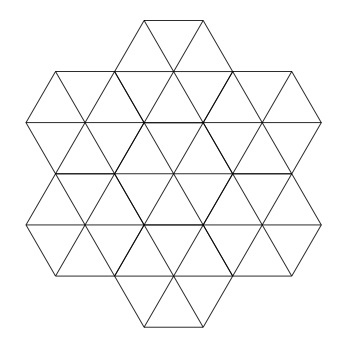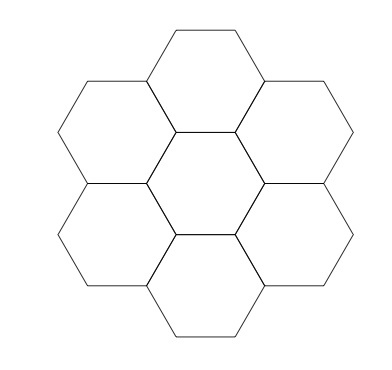# Regular Tessellations of the plane

Alignments to Content Standards: G-MG.A A-CED.A.2

A tessellation of the plane is an arrangement of polygons which cover the plane without gaps or overlapping. For example, part of a tessellation with rectangles is pictured below:A tessellation is called regular if all polygons in the tessellation are congruent regular polygons and if any two polygons in the tessellation either do not meet, share a vertex only, or share one edge. The checkerboard pattern below is an example of a regular tessellation which can be continued indefinitely in all directions:In this problem you will discover some very strong restrictions on possible tesselations of the plane, stemming from the fact that that each interior angle of an $n$ sided regular polygon measures $\frac{180(n-2)}{n}$ degrees.

1. Suppose $P_n$ is a regular $n$ sided polygon and there is a tessellation of the plane by polygons congruent to $P_n$. Suppose that $m$ of these polygons meet at each vertex in the tessellation. Explain why $$m \times \left(\frac{180(n-2)}{n}\right) = 360.$$
2. Show that for any such tesselation, we must have $m \geq 3$ and, using part (a), that $n \leq 6$.
3. Using (a) and (b), find all possible pairs $(m,n)$ for a regular tessellation of the plane. For each possibility, draw a picture of the corresponding tessellation.

## IM Commentary

This task examines the ways in which the plane can be covered by regular polygons in a very strict arrangement called a regular tessellation. These tessellations are studied here using algebra, which enters the picture via the formula for the measure of the interior angles of a regular polygon (which should therefore be introduced or reviewed before beginning the task). The goal of the task is to use algebra in order to understand which tessellations of the plane with regular polygons are possible. The regular tessellation with squares will be familiar to students from playing checkers, chess, and tic-tac-toe but also from observing the way square tiles are laid on both floors and ceilings. The hexagonal pattern is perhaps less familiar but it comes up in nature in many ways (molecular structure of graphite and structure of beehives for example). The triangular tessellation and hexagonal tessellation are closely linked since it is possible to get from the triangular tessellation to the hexagonal one by joining triangles at each vertex and from the hexagonal tessellation to the triangular one by adding centers of the hexagons and drawing lines to each vertex.

The modelling aspect and discovery-centric nature of experimenting with tessellations likely restricts this task for use only toward instructional purposes. In particular, students, perhaps in groups, should be encouraged to produce their own (non-regular) tessellations of the plane. If pattern tiles or other manipulatives are available this will be helpful. Some examination of the many extraordinary patterns in the work of M.C. Escher would also be rewarding to consider, though of course these tessellations use non-polygonal shapes.

## Solutions

Solution: 1

1. We are given that the interior angles of $P_n$ each measure $\frac{180 \times (n-2)}{n}$ degrees. Since there are $360$ degrees in a circle, if $m$ regular $n$ sided polygons fit together at a single vertex this means that $$m \times \left(\frac{180 \times (n-2)}{n}\right) = 360.$$

2. The interior angles of a regular polygon always measure less than $180$ degrees so two of them cannot come together and fill up the entire $360$ degrees around a vertex. This means that we need at least $3$ polygons meeting at each vertex and so $m \geq 3$.

We can rewrite the equation from part (a) as $$\frac{180 \times (n-2)}{n} = \frac{360}{m}.$$ We just saw that $m \geq 3$ and so the right hand side of this equation is at most $\frac{360}{3} = 120$. On the other hand, as $n$ grows, the left hand side of this equation gets closer and closer to $180$ and so this means that $n$ cannot be too large. More specifically, when $n = 6$ the left hand side fo the equation is equal to $120$ and once $n \gt 6$ it is larger than $120$. So we conclude that $n \leq 6$.

3. Since $n$ represents the number of sides of a regular polygon, we must have $n\geq 3$. We can check by hand the values $n = 3,4,5,6$ to see if there are solutions to the equations $$\frac{180 \times (n-2)}{n} = \frac{360}{m}.$$ When $n = 3$ we find $m = 6$. Whe $n = 4$ we find $m = 4$, When $n = 5$ we see that $m$ is not a whole number. But $m$ is the number of polygons meeting at a vertex so it must be a whole number if these polygons give a tesselation of the plane. Finally when $n = 6$ we have $m = 3$.

So our work gives three possibilities for regular tesselations of the plane. When $n = 3$ this would be a tesselation by equilateral triangles with $6$ triangles meeting at each vertex. This is possible and part of the tesselation is pictured below:When $n = 4$ we have $m = 4$ and this is the tesselation by squares part of which is pictured in the problem statement:Finally when $n = 6$ and $m = 3$ this is a tesselation by regular hexagons with three hexagons meeting at each vertex:Solution: 2 Alternate solution for part b

We start by dividing the equation from part (b) by $180$ and then multiplying by $n$ to find $$m \times (n-2) = 2n.$$ This means that $mn -2m -2n = 0$ or equivalently $(n-2) \times (m-2) -4 = 0$. So we find $$(n−2)(m−2)=4.$$ Note that $m \geq 3$ because at least three polygons are needed to fill in the full $360^\circ$ of a circle. Moreover $n \geq 3$ because a polygon must have at least $3$ sides. We can find all solutions to the above equation because the only possible factorizations of $4$ are $4 \times 1$ (corresponding to $n = 6, m = 3$), $2 \times 2$ (corresponding to $n = m = 4$), and $1 \times 4$ (corresponding to $n = 3$ and $m = 6$.